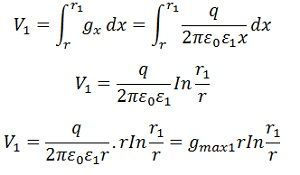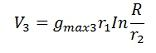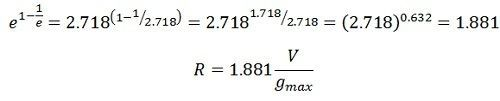Definition:Grading is defined as the process of equalizing the stress in the dielectric of the cable. Generally, the electrical stress is maximum at the surface of the conductor or the innermost part of conductor while it is minimum at the outermost sheath of the conductor. If the stress is equal to all the dielectric of the conductor, then the thickness of the conductor is reduced. But if the stress is maximum at any of the dielectrics then it increases the thickness of the cable due to which the cost of the cable also increases. There are two methods of grading the cable

In this type of grading, the homogeneous dielectric is replaced by layers of dielectric having a different value of relative permittivity. For getting a uniform stress, an infinite number of dielectric will be required. The electrical stress can be uniformly distributed by using two or more dielectric having suitable permittivity.The dielectric stress is given by the equationLet us considered a cable having three dielectrics of relative permittivity ε1, ε2, and ε3, such that ε1< ε2< ε3. Let r1, r2 and R be the outer radii of the dielectric.

The potential difference across the inner layer isSimilarly the potential difference between r1 and r2 i.e., across the middle layerand the potential difference between r2 and R i.e., across the outer layerThe total potential difference between core and earthed sheathIn case the maximum stress is the same in the each layerThe total voltage applied across the cablegmax represent the peak value of electrical stress, and all the voltages are represented in peak values, not in RMS value.

Intersheath grading is the method of keeping the gradual voltage across the insulator by using the layers of the insulators. In this method, the uniform voltage is developed across the cable insulators. The total layer of the insulation material is divided into numbers of layers by providing intersheath.

Intersheaths are thin metallic cylindrical sheaths concentric with the conductor and placed between the conductor and the outside sheath. Consider a cable with one intersheath only as shown below.Consider a cable with one intersheath only as shown in the figure below.

Let r1= radius of the intersheath
V1 = voltage between the core and the intersheath
V2= voltage between the innersheath and outer sheath
V = applied voltage between the core and the sheath

The maximum potential gradient in the second layerThe maximum potential gradient in second equationIf the two potential gradients are equalWhere A is a constant equal to 1/gmax e 1-1/e

For minimum value of R

###Limitations of Grading

The main disadvantage of the capacitance grading is that the range of permittivity value of insulating material available for cable insulation is limited. The permittivity of the layers may not remain constant thereby change the stress distribution and cause the insulation break down at normal operating condition.

In Intershaeth grading, the intersheath layers are very thin and are liable to be damaged during transportation or installation. Also, thin intersheath are not able to carry the damage charging current of long cable line and thus the current-carrying capacity of the cable is reduced. For these reasons the present trends is to avoid grading